Magical Mathematics
Developer(s)Sachen
Publisher(s)Thin Chen Enterprise
Year released1990
System(s)NES, Q-Boy
Genre(s) Education Single player
Links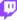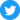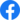Magical Mathematics is a math-based game for the NES. You can play in various modes, solving equations which focus on different mathematical operations. There is also a tile-clearing mini-game. This game was re-released in the Mindblower Pak as Math Quiz, along with Arctic Adventure, Jackpot and Galactic Crusader.

## Getting started

Press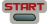at the start screen, or watch demos of the games. Pressat any time during a demo to return to the start screen. Once you're ready, you will go to the game selection page. Use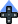and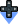to highlight the different options, andto choose one.

## Games

All games except for Mathematic Blocks is played in the game screen consisting of the title of the game, an area for the question, an area for the explanation should you get an answer wrong, a section for your answer and the keyboard where you type in your response. Also, across the top of the answer area is a countdown timer, the number of questions asked of you, and the number right and wrong answers you've given so far.

You have 2:00 minutes to answer each question. Type the answer with the keyboard on the lower right by selecting the key with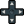and confirming withor pressing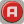on the = button. Possible operations include addition, subtraction, multiplication and division. Negative numbers are used and order of operations can be modified with parentheses. Use the N key to specify the numerator of a fraction before inputting the number, and use the N key for the denominator.

### Integral

Basic arithmetic with integers (whole numbers from negative infinity to positive infinity).

Example:

$2+(-2)\times 2=?$### Fraction

Basic arithmetic with integers and fractions. Answers must be fully reduced.

Example:

${\frac {8}{5}}/{\frac {-14}{15}}=?$### Equation with one unknown

Basic algebra solving for x.

Examples:

$10x+12\times (x-2)=108,x=?$${\frac {x}{2}}+1=5,x=?$### Compound Exercise

Solve the equations using a combination of the first three games.

Examples:

$(27+12)\times (-7)-54=?$${\frac {x}{6}}-{\frac {x}{9}}=1,x=?$### Mathematic Blocks

This is the only real game within the title. The aim is to remove all blocks from the playing field. You can remove 2 blocks at the same time, when they equal the equation on the right. For example $?+?=9$means you can remove a "4" block and a "5" block because $4+5=9$. You can only use the blocks if they have a direct link to each other. You cannot, however, remove 2 blocks that are just next to each other. Make sure you get the right order for the equations, + doesn't matter but - maybe…

You have 5:00 minutes to finish a level. Running out of time does not mean you lose straight away. But you will need to have removed a good part of the blocks by then. If you haven't cleared enough blocks you will get a message saying, "Too many lost!!!"

For each combination you get 100 points. If you attempt to remove two blocks that can't be removed at this time, or do not match an equation, you lose 50 points. Your high score will be displayed at the end of the game.